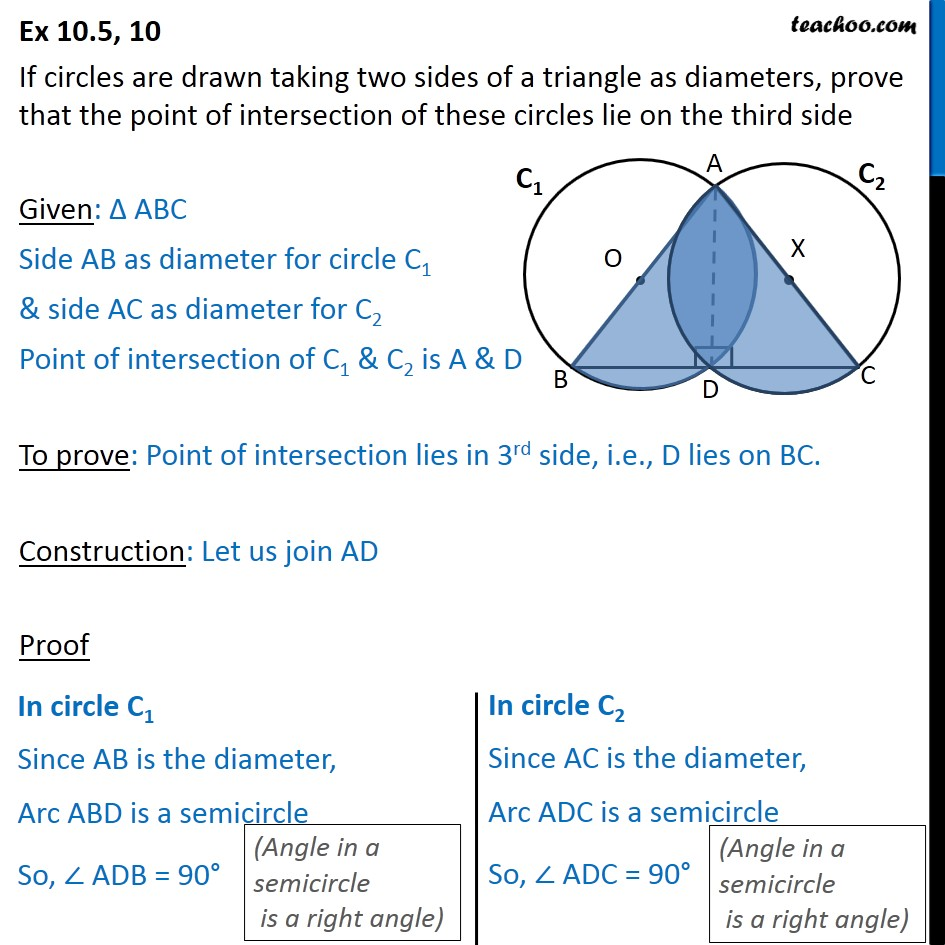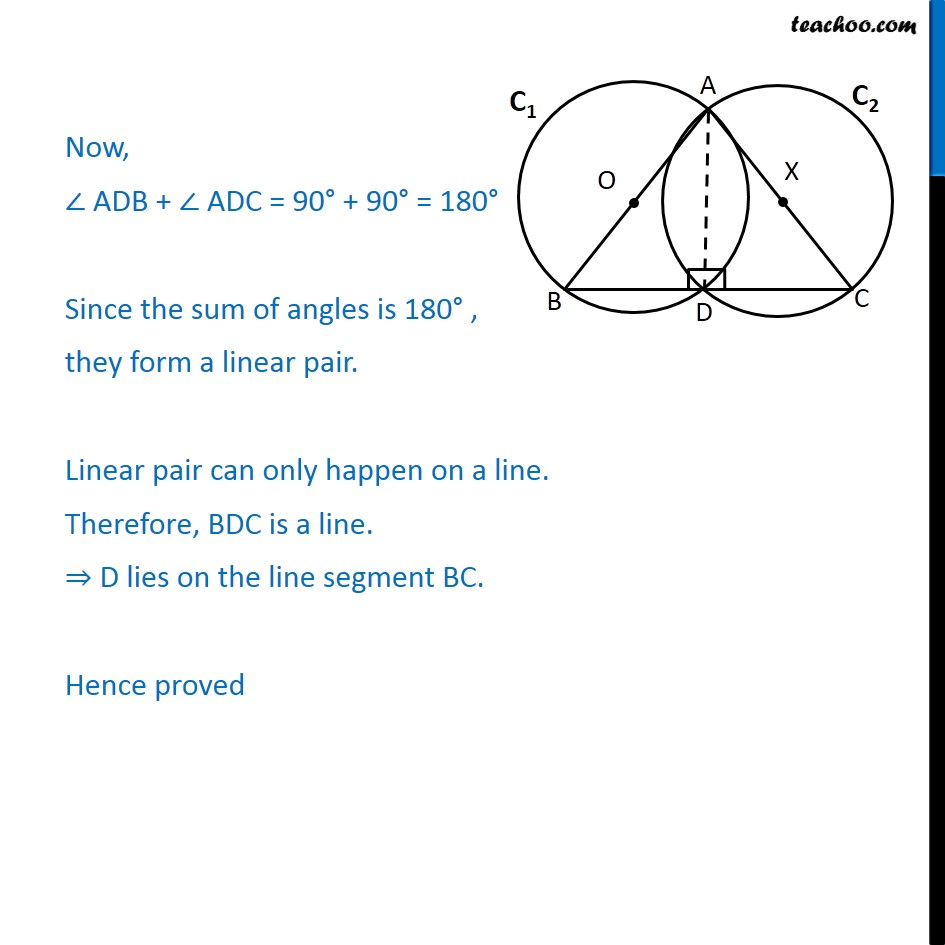Ex 10.5

Chapter 10 Class 9 Circles
Serial order wiseGet live Maths 1-on-1 Classs - Class 6 to 12

### Transcript

Ex 10.5, 10 If circles are drawn taking two sides of a triangle as diameters, prove that the point of intersection of these circles lie on the third side Given: ABC Side AB as diameter for circle C1 & side AC as diameter for C2 Point of intersection of C1 & C2 is A & D To prove: Point of intersection lies in 3rd side, i.e., D lies on BC. Construction: Let us join AD Proof Now, ADB + ADC = 90 + 90 = 180 Since the sum of angles is 180 , they form a linear pair. Linear pair can only happen on a line. Therefore, BDC is a line. D lies on the line segment BC. Hence proved